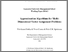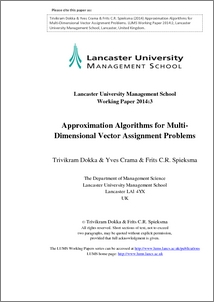# Approximation algorithms for multi-dimensional vector assignment problems

Dokka, Trivikram and Crama, Yves and Spieksma, Frits (2014) Approximation algorithms for multi-dimensional vector assignment problems. Working Paper. Lancaster University, Lancaster.Preview
PDF (TDokka_DO)
TDokka_DO.pdf - Published Version

## Abstract

We consider a special class of axial multi-dimensional assignment problems called multi-dimensional vector assignment (MVA) problems. An instance of the MVA problem is defined by m disjoint sets, each of which contains the same number n of p-dimensional vectors with nonnegative integral components, and a cost function defined on vectors.The cost of an m-tuple of vectors is defined as the cost of their component-wise maximum. The problem is now to partition the m sets of vectors into n m-tuples so that no two vectors from the same set are in the same m-tuple and so that the total cost of the m-tuples is minimized. The main motivation comes from a yield optimization problem in semi-conductor manufacturing. We consider two classes of polynomial-time heuristics for MVA, namely, hub heuristics and sequential heuristics, and we study their approximation ratio. In particular, we show that when the cost function is monotone and subadditive, hub heuristics, as well as sequential heuristics, have finite approximation ratio for every fixed m. Moreover, we establish better approximation ratios for certain variants of hub heuristics and sequential heuristics when the cost function is monotone and submodular, or when it is additive. We provide examples to illustrate the tightness of our analysis. Furthermore, we show that the MVA problem is APX-hard even for the case m = 3 and for binary input vectors. Finally, we show that the problem can be solved in polynomial time in the special case of binary vectors with fixed dimension p.

Item Type:
Monograph (Working Paper)
Departments:
ID Code:
69220
Deposited By:
Deposited On:
24 Apr 2014 15:05
Refereed?:
No
Published?:
Published